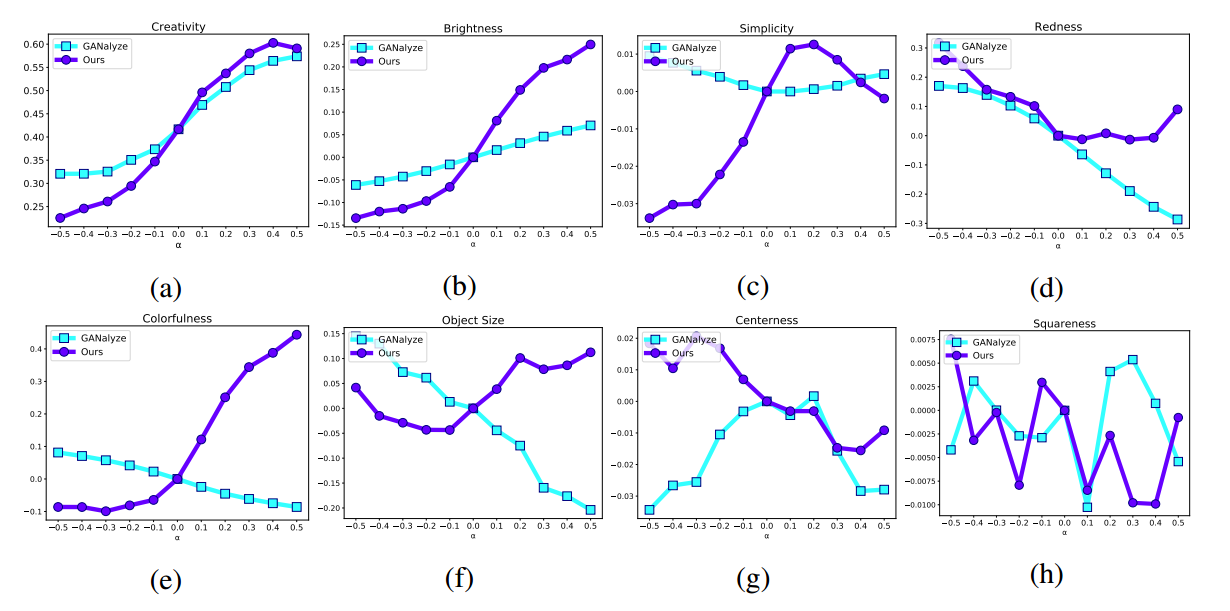# Exploring Latent Dimensions of Crowd-sourced Creativity

Bogazici University

## Abstract

Recently, the discovery of interpretable directions in the latent spaces of pre-trained GANs has become a popular topic. While existing works mostly consider directions for semantic image manipulations, we focus on an abstract property: creativity. Can we manipulate an image to be more or less creative? We build our work on the largest AI-based creativity platform, Artbreeder, where users can generate images using pre-trained GAN models. We explore the latent dimensions of images generated on this platform and present a novel framework for manipulating images to make them more creative. Our code and dataset are available in this repository.

## Introduction

One of the most popular platforms that collaboratively take advantage of generative adversarial networks is Artbreeder. Artbreeder helps users create new images using a BigGAN model, where users can adjust parameters or mix different images to generate new ones. Artbreeder platform is investigated by several recent work. In this study, we explore the manipulation of the latent space for creativity using a large dataset from the Artbreeder platform. We use this data as a proxy to build an assessor model that indicates the extent of creativity for a given image. Our approach modifies the image semantics by shifting the latent code towards creativity by a certain amount to make it more or less creative.

## Methodology

In this paper, we use a pre-trained BigGAN model to manipulate images towards creativity and build our work on the GANalyze model. Given a generator G, a class vector y, a noise vector z, and an assessor function A, the GANalyze model solves the following problem: $$\mathcal{L}(\theta) = \mathbb{E}_{\mathbf{z},\mathbf{y},\alpha} [ A(G(T_{\theta}(\mathbf{z}, \alpha), \mathbf{y})) - (A(G(\mathbf{z}, \mathbf{y})) + \alpha))^2 ]$$ where α is a scalar value representing the degree of manipulation, θ is the desired direction, and T is the transformation function defined as $$T_\theta (\mathbf{z},\alpha) = \mathbf{z} + \alpha\theta$$ that moves the input z along the direction θ. In this work, we extend the GANalyze framework where we use a neural network that uses noise drawn from a distribution N (0; 1) which learns to map an input to different but functionally related (e.g., more creative) outputs: $$\mathcal{L}(\theta) = \mathbb{E}_{\mathbf{z},\mathbf{y},\alpha} [ (A(G(F_{z}(\mathbf{z}, \alpha), F_{y}(\mathbf{y}, \alpha)))) - (A(G(\mathbf{z}, \mathbf{y})) + \alpha))^2 ]$$ where the first term represents the score of the modified image after applying the function F with parameters z, y, and α, and the second term simply represents the score of the original image increased or decreased by α. Fz computes a diverse direction θ with a noise ϵ as $$F_{z}(\mathbf{z}, \alpha) = \mathbf{z} + \alpha \cdot \mathbf{NN}(\mathbf{z}, \epsilon)$$ Moreover, we also learn a direction for class vectors as follows: $$\mathcal{L}(\theta) = \mathbb{E}_{\mathbf{z},\mathbf{y},\alpha} [ A(G(F_{z}(\mathbf{z}, \alpha), F_{y}(\mathbf{y}, \alpha))) - (A(G(\mathbf{z}, \mathbf{y})) + \alpha))^2 ]$$ where Fy is calculated using a 2-layer neural network (NN) as follows $$F_{y}(\mathbf{y}, \alpha) = \mathbf{y} + \alpha \cdot \mathbf{NN}(\mathbf{y}, \epsilon)$$

## Results

We first investigate whether our model learns to navigate the latent space in such a way that it can increase or decrease creativity by a given α value. As can be seen from Figure 1 (a), our model achieves a much lower creativity score for low α values such as α = −0:5, while simultaneously achieving higher creativity for α = 0:4 and α = 0:5 compared to GANalyze. Next, we investigate what kind of image factors are changed to achieve these improvements.Figure 1: Our method learns a better manipulation of the latent space compared to GANalyze. For each figure, the x-axis represents the α-value and the y-axis represents the mean.

Following GANalyze, we examined redness, colorfulness, brightness, simplicity, squareness, centeredness and object size. We observe that both methods follow similar trends except colorfulness and object size. In particular, our method prefers to increase colorfulness and object size with increasing α values. Figure 2 shows some of the samples generated by our method and GANalyze. As can be seen from the visual results, our method is capable of performing several diverse manipulations on the input images.

• Redness: is calculated as the normalized number of red pixels.
• Colorfulness: is calculated by the metric from this paper.
• Brightness: is calculated as the average pixel value after the grayscale transformation.
• Simplicity: is computed as a histogram of pixel intensity used to measure entropy.
• Object Size: Following GANalyze, we used Mask R-CNN to create a segmentation mask at the instance level of the generated image. For Object Size, we used the difference in the mask area when the α value increases or decreases.
• Centeredness: is calculated as the deviation between the centroid of the mask from the center of the frame.
• Squareness: is calculated as the ratio of the length of the minor and major axes of an ellipse with the same normalized central moments as the mask.

## Conclusion and Limitations

Our method is limited to manipulating GAN-generated images. As with any image synthesis tool, our method faces similar concerns and dangers of misuse if it can be applied to images of people or faces for malicious purposes, as described in this paper. Our work also sheds light on the understanding of creativity and opens up possibilities to improve human-made art through GAN applications. On the other hand, we point out that using GAN-generated images as a proxy for creativity may bring its own limitations.

## BibTeX

@article{kocasari2021latentcreative,
author    = {Kocasari, Umut and Bag, Alperen and Atici, Efehan and Yanardag, Pinar},
title     = {Exploring Latent Dimensions of Crowd-sourced Creativity},
journal   = {NeurIPS 2021 Workshop on Machine Learning for Creativity and Design},
year      = {2021},
}